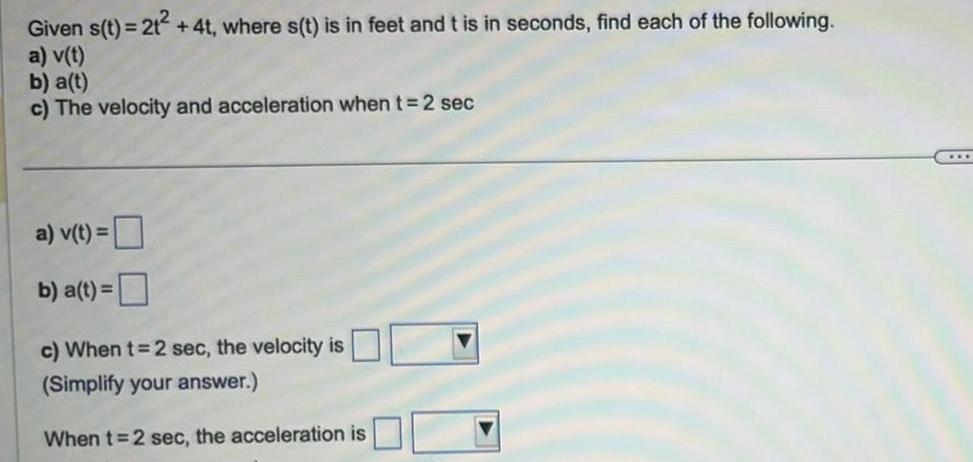Question:

# Given s t 2t 4t where s t is in feet and t is in seconds

Last updated: 9/20/2023Given s t 2t 4t where s t is in feet and t is in seconds find each of the following a v t b a t c The velocity and acceleration when t 2 sec a v t b a t c When t 2 sec the velocity is Simplify your answer When t 2 sec the acceleration is